## Huffman Coding-

• Huffman Coding is a famous Greedy Algorithm.
• It is used for the lossless compression of data.
• It uses variable length encoding.
• It assigns variable length code to all the characters.
• The code length of a character depends on how frequently it occurs in the given text.
• The character which occurs most frequently gets the smallest code.
• The character which occurs least frequently gets the largest code.
• It is also known as Huffman Encoding.

## Prefix Rule-

• Huffman Coding implements a rule known as a prefix rule.
• This is to prevent the ambiguities while decoding.
• It ensures that the code assigned to any character is not a prefix of the code assigned to any other character.

## Major Steps in Huffman Coding-

There are two major steps in Huffman Coding-

1. Building a Huffman Tree from the input characters.
2. Assigning code to the characters by traversing the Huffman Tree.

## Huffman Tree-

The steps involved in the construction of Huffman Tree are as follows-

### Step-01:

• Create a leaf node for each character of the text.
• Leaf node of a character contains the occurring frequency of that character.

### Step-02:

• Arrange all the nodes in increasing order of their frequency value.

### Step-03:

Considering the first two nodes having minimum frequency,

• Create a new internal node.
• The frequency of this new node is the sum of frequency of those two nodes.
• Make the first node as a left child and the other node as a right child of the newly created node.

### Step-04:

• Keep repeating Step-02 and Step-03 until all the nodes form a single tree.
• The tree finally obtained is the desired Huffman Tree.

## Time Complexity-

The time complexity analysis of Huffman Coding is as follows-

• extractMin( ) is called 2 x (n-1) times if there are n nodes.
• As extractMin( ) calls minHeapify( ), it takes O(logn) time.

Thus, Overall time complexity of Huffman Coding becomes O(nlogn).

Here, n is the number of unique characters in the given text.

## Important Formulas-

The following 2 formulas are important to solve the problems based on Huffman Coding-

### Formula-01: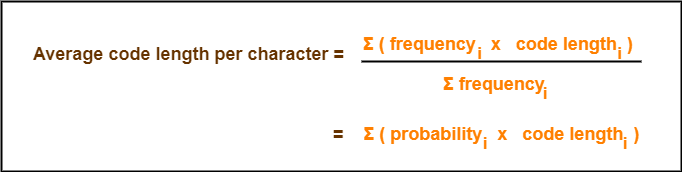### Formula-02:

Total number of bits in Huffman encoded message

= Total number of characters in the message x Average code length per character

= ∑ ( frequencyi x Code lengthi )

## Problem-

A file contains the following characters with the frequencies as shown. If Huffman Coding is used for data compression, determine-

1. Huffman Code for each character
2. Average code length
3. Length of Huffman encoded message (in bits)

 Characters Frequencies a 10 e 15 i 12 o 3 u 4 s 13 t 1

## Solution-

First let us construct the Huffman Tree.

Huffman Tree is constructed in the following steps-

### Step-01: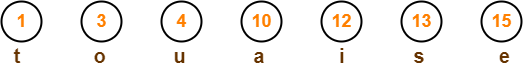### Step-02: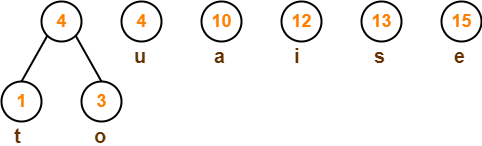### Step-03: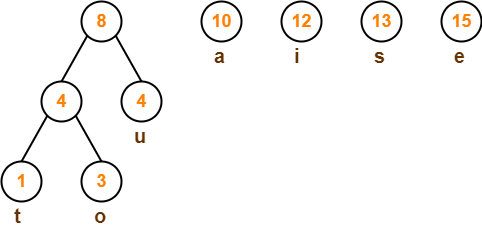### Step-04: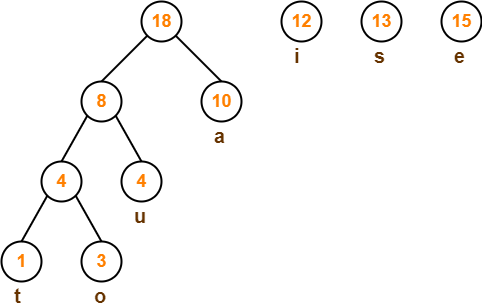### Step-05: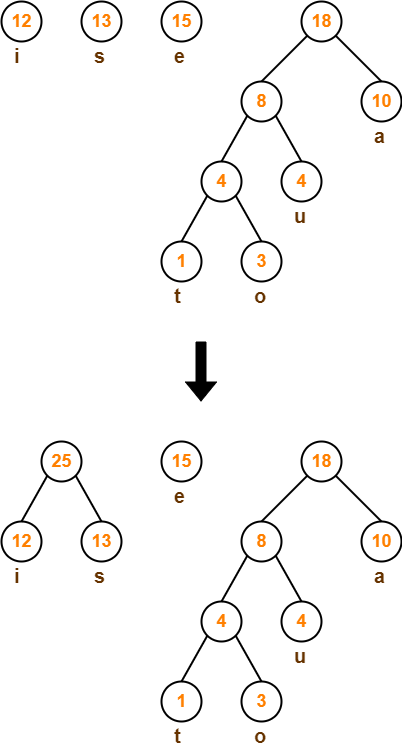### Step-06: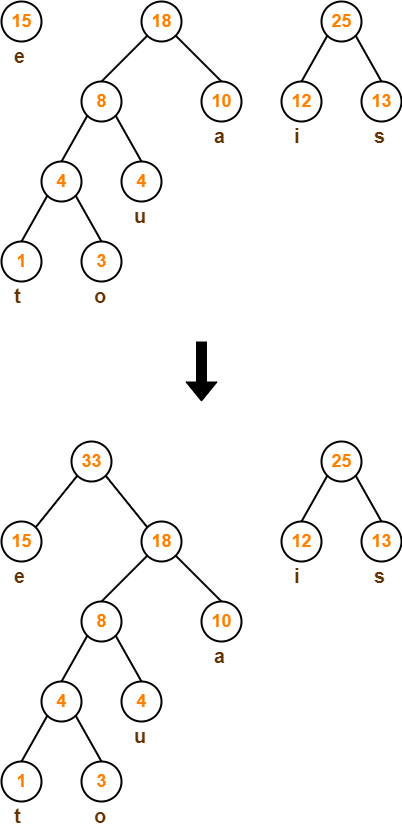### Step-07: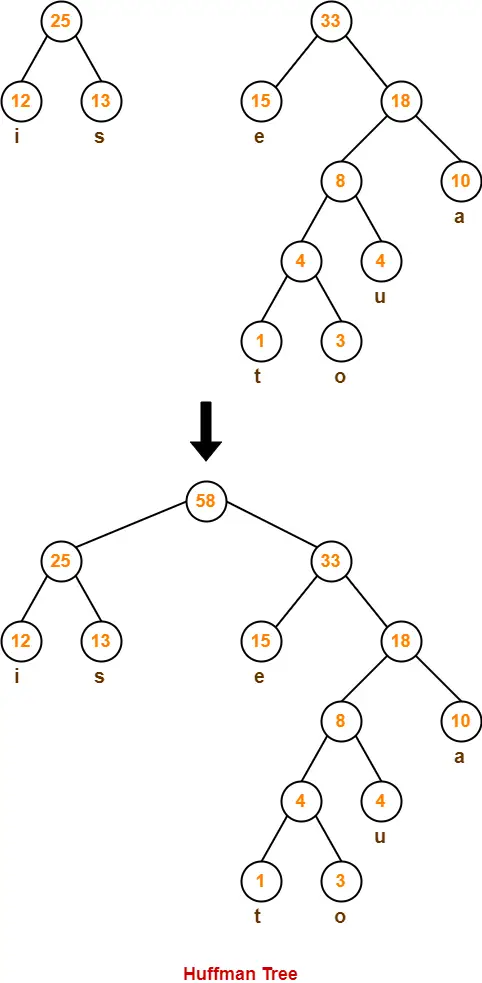Now,

• We assign weight to all the edges of the constructed Huffman Tree.
• Let us assign weight ‘0’ to the left edges and weight ‘1’ to the right edges.

### Rule

• If you assign weight ‘0’ to the left edges, then assign weight ‘1’ to the right edges.
• If you assign weight ‘1’ to the left edges, then assign weight ‘0’ to the right edges.
• Any of the above two conventions may be followed.
• But follow the same convention at the time of decoding that is adopted at the time of encoding.

After assigning weight to all the edges, the modified Huffman Tree is-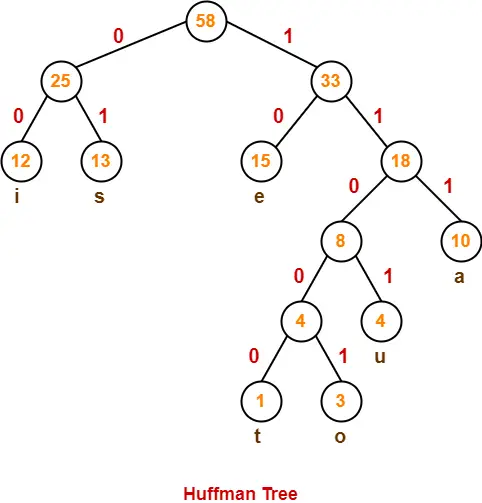Now, let us answer each part of the given problem one by one-

### 1. Huffman Code For Characters-

To write Huffman Code for any character, traverse the Huffman Tree from root node to the leaf node of that character.

Following this rule, the Huffman Code for each character is-

• a = 111
• e = 10
• i = 00
• o = 11001
• u = 1101
• s = 01
• t = 11000

From here, we can observe-

• Characters occurring less frequently in the text are assigned the larger code.
• Characters occurring more frequently in the text are assigned the smaller code.

### 2. Average Code Length-

Using formula-01, we have-

Average code length

= ∑ ( frequencyi x code lengthi ) / ∑ ( frequencyi )

= { (10 x 3) + (15 x 2) + (12 x 2) + (3 x 5) + (4 x 4) + (13 x 2) + (1 x 5) } / (10 + 15 + 12 + 3 + 4 + 13 + 1)

= 2.52

### 3. Length of Huffman Encoded Message-

Using formula-02, we have-

Total number of bits in Huffman encoded message

= Total number of characters in the message x Average code length per character

= 58 x 2.52

= 146.16

≅ 147 bits

To gain better understanding about Huffman Coding,

Watch this Video Lecture

To practice previous years GATE problems on Huffman Coding,

Watch this Video Lecture

Next Article- 0/1 Knapsack Problem

Get more notes and other study material of Design and Analysis of Algorithms.

Watch video lectures by visiting our YouTube channel LearnVidFun.